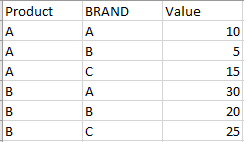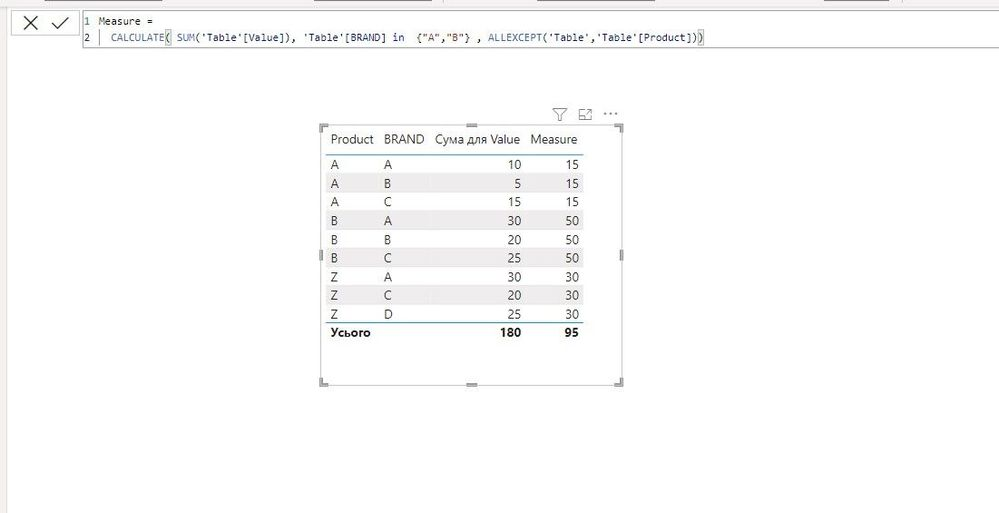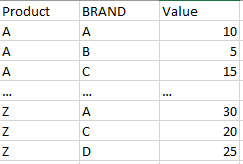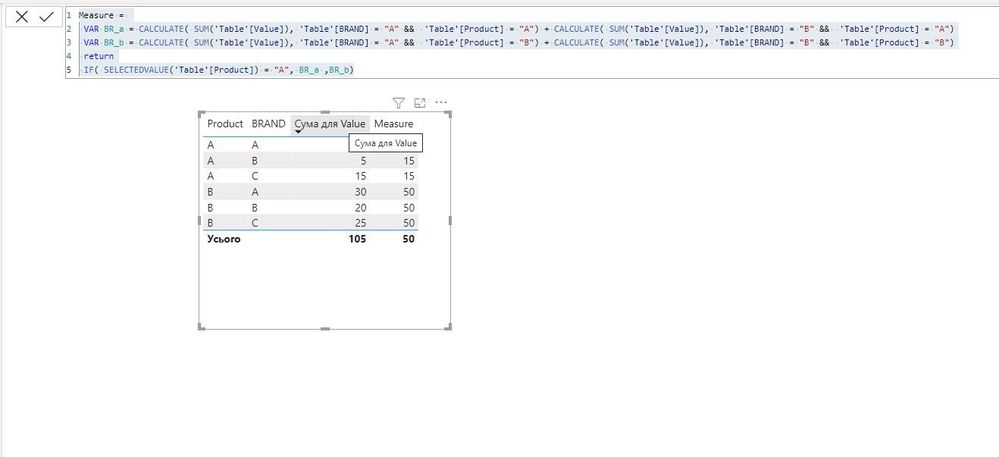cancel
Showing results for
Did you mean:New Member

## Create a new total column per specific row

Hello,

I need some help for summarize row in a new column with a condition on rows

The logic is : Sum for each product the value of BRAND A & BRAND B and put in every row

What i have :What i want :Sum of product A BRAND A + product A BRAND B = 15

Sum of product B BRAND A + product B BRAND B = 50

1 ACCEPTED SOLUTIONSuper User

@ValentintT Then try this Measure, also attached an example file

``````Measure =
CALCULATE( SUM('Table'[Value]), 'Table'[BRAND] in  {"A","B"} , ALLEXCEPT('Table','Table'[Product]))``````__________________________________________

Thank you for your like and decision

__________________________________________

Greetings from Ukraine

To help me grow PayPal: embirddima@gmail.com
5 REPLIES 5New Member

But i expressed my problem badly
I need it for every products but A and B aren't the only products :Super User

@ValentintT Then try this Measure, also attached an example file

``````Measure =
CALCULATE( SUM('Table'[Value]), 'Table'[BRAND] in  {"A","B"} , ALLEXCEPT('Table','Table'[Product]))``````__________________________________________

Thank you for your like and decision

__________________________________________

Greetings from Ukraine

To help me grow PayPal: embirddima@gmail.comNew Member

It's perfect ! thanks for this solutionSuper User

@ValentintT How many products do you have in the table?

__________________________________________

Thank you for your like and decision

__________________________________________

Greetings from Ukraine

To help me grow PayPal: embirddima@gmail.comSuper User

Hi @ValentintT try it

``````Measure =
VAR BR_a = CALCULATE( SUM('Table'[Value]), 'Table'[BRAND] = "A" &&  'Table'[Product] = "A") + CALCULATE( SUM('Table'[Value]), 'Table'[BRAND] = "B" &&  'Table'[Product] = "A")
VAR BR_b = CALCULATE( SUM('Table'[Value]), 'Table'[BRAND] = "A" &&  'Table'[Product] = "B") + CALCULATE( SUM('Table'[Value]), 'Table'[BRAND] = "B" &&  'Table'[Product] = "B")
return
IF( SELECTEDVALUE('Table'[Product]) = "A", BR_a ,BR_b)``````__________________________________________

Thank you for your like and decision

__________________________________________

Greetings from Ukraine

To help me grow PayPal: embirddima@gmail.com